ICSE Class 9 Isosceles Triangle Solution New Pattern

## ICSE Class 9 Isosceles Triangle Solution New Pattern By Clarify Knowledge

ICSE Class 9 Isosceles Triangle Solution New Pattern 2022

## Chapter 10 - Isosceles Triangle Exercise Ex. 10(A)

Question 1

In the figure alongside,

AB = AC

A = 48o and

ACD = 18o.

Show that BC = CD.Solution 1

In

BAC + ACB + ABC = 1800

480 + ACB + ABC = 1800

But ACB = ABC [AB = AC]

2ABC = 1800 - 480

2ABC = 1320

ABC = 660 = ACB ……(i)

ACB = 660

ACD + DCB = 660

180 + DCB = 660

DCB = 480 ………(ii)

Now, In

DBC = 660 [From (i), Since ABC = DBC]

DCB = 48[From (ii)]

BDC = 1800 - 480 - 660

BDC = 660

Since BDC = DBC

Therefore, BC = CD

Equal angles have equal sides opposite to them.Question 2

Calculate:

(ii) ABC

(iii) BAC

Solution 2

Given: ACE = 1300; AD = BD = CD

Proof:

(i)

(ii)

(iii)

Question 3

In the following figure, AB = AC; BC = CD and DE is parallel to BC. Calculate:

(i) CDE

(ii) DCE

Solution 3

(i)

(ii)

Question 4

Calculate x:

(i)

(ii)

Solution 4

(i) Let the triangle be ABC and the altitude be AD.

(ii) Let triangle be ABC and altitude be AD.

Question 5

In the figure, given below, AB = AC. Prove that: BOC = ACD.

Solution 5

Let ABO =OBC = x and ACO = OCB = y

Now,

Question 6

In the figure given below, LM = LN; angle PLN = 110o. Calculate:

(i) LMN

(ii) MLNSolution 6

Given:

(i) We know that the sum of the measure of all the angles of a quadrilateral is 360o.

(ii)

Question 7

An isosceles triangle ABC has AC = BC. CD bisects AB at D and CAB = 55o.

Find: (i) DCB (ii) CBD.Solution 7

Now,

Question 8

Find x:

Solution 8

Let us name the figure as following:

For x:

Question 9

In the triangle ABC, BD bisects angle B and is perpendicular to AC. If the lengths of the sides of the triangle are expressed in terms of x and y as shown, find the values of x and y.

Solution 9

Therefore,

and AB = BC

Substituting the value of x from (i)

Putting y = 3 in (i)

x = 3 + 1

x = 4Question 10

In the given figure; AE // BD, AC // ED and AB = AC. Find a, b and c.

Solution 10

Let P and Q be the points as shown below:

Given:

Question 11

In the following figure; AC = CD, AD = BD and C = 58o.

Find angle CAB.Solution 11

Now,

Question 12

In the figure of q. no. 11 given above, if AC = AD = CD = BD; find angle ABC.

Solution 12

Question 13

In triangle ABC; AB = AC and A : B = 8 : 5; find angle A.Solution 13

Let

Given: AB = AC

[Angles opp. to equal sides are equal]

Question 14

In triangle ABC; A = 60oC = 40o, and bisector of angle ABC meets side AC at point P. Show that BP = CP.Solution 14

Now,

BP is the bisector of

Question 15

In triangle ABC; angle ABC = 90o and P is a point on AC such that PBC = PCB. Show that: PA = PB.Solution 15

Let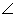PBC =PCB = x

In the right angled triangle ABC,

and

Therefore in the triangle ABP;

Hence,

PA = PB [sides opp. to equal angles are equal]Question 16

ABC is an equilateral triangle. Its side BC is produced upto point E such that C is mid-point of BE. Calculate the measure of angles ACE and AEC.Solution 16

Question 17

In triangle ABC, D is a point in AB such that AC = CD = DB. If ∠B = 28°, find the angle ACD.Solution 17

Question 18

In the given figure, AD = AB = AC, BD is parallel to CA and angle ACB = 65°. Find angle DAC.

Solution 18

Question 19

Prove that a triangle ABC is isosceles, if:

(i)  altitude AD bisects angles BAC, or

(ii)  bisector of angle BAC is perpendicular to base BC.Solution 19

(i)  In ΔABC, let the altitude AD bisects ∠BAC.

Then we have to prove that the ΔABC is isosceles.

⇒ AB = AC (cpct)

Hence, ΔABC is an isosceles.

(ii)  In Δ ABC, the bisector of ∠ BAC is perpendicular to the base BC. We have to prove that the ΔABC is isosceles.

⇒ AB = AC (cpct)

Hence, ΔABC is an isosceles.Question 20

In the given figure; AB = BC and AD = EC.

Prove that: BD = BE.

Solution 20

In ΔABC,

AB = BC (given)

⇒ ∠BCA = ∠BAC (Angles opposite to equal sides are equal)

⇒ ∠BCD = ∠BAE ….(i)

⇒ AD + DE = EC + DE (Adding DE on both sides)

⇒ AE = CD ….(ii)

Now, in triangles ABE and CBD,

AB = BC (given)

∠BAE = ∠BCD [From (i)]

AE = CD [From (ii)]

⇒ ΔABE ≅ ΔCBD

⇒ BE = BD (cpct)

## Chapter 10 - Isosceles Triangle Exercise Ex. 10(B)

Question 1

If the equal sides of an isosceles triangle are produced, prove that the exterior angles so formed are obtuse and equal.Solution 1

Const: AB is produced to D and AC is produced to E so that exterior angles  and  is formed.

Since angle B and angle C are acute they cannot be right angles or obtuse angles.

Now,

Therefore, exterior angles formed are obtuse and equal.Question 2

In the given figure, AB = AC. Prove that:

(i) DP = DQ

(ii) AP = AQ

(iii) AD bisects angle ASolution 2

(i)

(ii) We have already proved that

Therefore,BP = CQ[cpct]

Now,

AB = AC[Given]

AB - BP = AC - CQ

AP = AQ

(iii)

Hence, AD bisects angle A.Question 3

In triangle ABC, AB = AC; BE AC and CF AB. Prove that:

(i) BE = CF

(ii) AF = AESolution 3

(i)

(ii)Since

Question 4

In isosceles triangle ABC, AB = AC. The side BA is produced to D such that BA = AD. Prove that: BCD = 90oSolution 4

Const: Join CD.

Question 5

(i) In triangle ABC, AB = AC and = 36°. If the internal bisector of meets AB at point D, prove that AD = BC.

(ii) If the bisector of an angle of a triangle bisects the opposite side, prove that the triangle is isosceles.Solution 5

Question 6

Prove that the bisectors of the base angles of an isosceles triangle are equal.Solution 6

Question 7

In the given figure, AB = AC and DBC = ECB = 90o

Prove that:

(i) BD = CE

DBC = ECB = 90o[Given]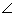DBC =ECB …….(ii)

Subtracting (i) from (ii)

Question 8

ABC and DBC are two isosceles triangles on the same side of BC. Prove that:

(i) DA (or AD) produced bisects BC at right angle.

(ii) BDA = CDA.Solution 8

DA is produced to meet BC in L.

Subtracting (i) from (ii)

From (iii), (iv) and (v)

From (vi) and (vii)

Now,

Question 9

The bisectors of the equal angles B and C of an isosceles triangle ABC meet at O. Prove that AO bisects angle A.Solution 9

In ABC, we have AB = AC

B = C [angles opposite to equal sides are equal]

Now,

In ABO and ACO,

AB = AC [Given]

OBC = OCB [From (i)]

OB = OC [From (ii)]

Therefore, AO bisects BAC.Question 10

Prove that the medians corresponding to equal sides of an isosceles triangle are equal.Solution 10

Question 11

Use the given figure to prove that, AB = AC.

Solution 11

From (i), (ii) and (iii)

Question 12

In the given figure; AE bisects exterior angle CAD and AE is parallel to BC.

Prove that: AB = AC.

Solution 12

Since AE || BC and DAB is the transversal

Since AE || BC and AC is the transversal

But AE bisects

AB = AC[Sides opposite to equal angles are equal]Question 13

In an equilateral triangle ABC; points P, Q and R are taken on the sides AB, BC and CA respectively such that AP = BQ = CR. Prove that triangle PQR is equilateral.Solution 13

AB = BC = CA…….(i) [Given]

AP = BQ = CR…….(ii) [Given]

Subtracting (ii) from (i)

AB - AP = BC - BQ = CA - CR

BP = CQ = AR …………(iii)

……..(iv) [angles opp. to equal sides are equal]

From (v) and (vi)

PQ = QR = PR

Therefore, PQR is an equilateral triangle.Question 14

In triangle ABC, altitudes BE and CF are equal. Prove that the triangle is isosceles.Solution 14

In ABE and ACF,

A = A[Common]

AEB = AFC = 900[Given: BE AC; CF AB]

BE = CF[Given]

Therefore, ABC is an isosceles triangle.Question 15

Through any point in the bisector of angle, a straight line is drawn parallel to either arm of the angle. Prove that the triangle so formed is isosceles.Solution 15

AL is bisector of angle A. Let D is any point on AL. From D, a straight line DE is drawn parallel to AC.

DE || AC [Given]

DAC = DAE…….(ii) [AL is bisector of A]

From (i) and (ii)

AE = ED [Sides opposite to equal angles are equal]

Therefore, AED is an isosceles triangle.Question 16

In triangle ABC; AB = AC. P, Q and R are mid-points of sides AB, AC and BC respectively. Prove that:

(i) PR = QR(ii) BQ = CPSolution 16

(i)

In ABC,

AB = AC

AP = AQ …….(i)[ Since P and Q are mid - points]

In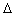BCA,

PR = [PR is line joining the mid - points of AB and BC]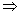PR = AQ……..(ii)

InCAB,

QR = [QR is line joining the mid - points of AC and BC]QR = AP……(iii)

From (i), (ii) and (iii)

PR = QR

(ii)

AB = AC

B = C

Also,

In BPC and CQB,

BP = CQB =C

BC = BC

Therefore, ΔBPCΔCQB   [SAS]

BP = CPQuestion 17

From the following figure, prove that:

(i) ACD = CBE

Solution 17

(i) In ACB,

AC = AC[Given]

ABC = ACB …….(i)[angles opposite to equal sides are equal]

ACD + ACB = 1800 …….(ii)[DCB is a straight line]

ABC + CBE = 1800 ……..(iii)[ABE is a straight line]

Equating (ii) and (iii)ACD +ACB =ABC +CBEACD +ACB =ACB +CBE[From (i)]ACD =CBE

(ii)

Question 18

Equal sides AB and AC of an isosceles triangle ABC are produced. The bisectors of the exterior angle so formed meet at D. Prove that AD bisects angle A.Solution 18

AB is produced to E and AC is produced to F. BD is bisector of angle CBE and CD is bisector of angle BCF. BD and CD meet at D.

In ABC,

AB = AC[Given]

C = B[angles opposite to equal sides are equal]

CBE = 1800 - B[ABE is a straight line]

[BD is bisector of CBE]

Similarly,

BCF = 1800 - C[ACF is a straight line]

[CD is bisector of BCF]

Now,

In BCD,

BD = CD

In ABD and ACD,

AB = AC[Given]

BD = CD[Proved]

ABC is a triangle. The bisector of the angle BCA meets AB in X. A point Y lies on CX such that AX = AY.

Prove that CAY = ABC.Solution 19

In ABC,

CX is the angle bisector of C

ACY = BCX ....... (i)

In AXY,

AX = AY [Given]

AXY = AYX …….(ii) [angles opposite to equal sides are equal]

Now XYC = AXB = 180° [straight line]

AYX + AYC = AXY + BXY

AYC = BXY ........ (iii) [From (ii)]

In AYC and BXC

AYC + ACY + CAY = BXC + BCX + XBC = 180°

CAY = XBC [From (i) and (iii)]

CAY = ABCQuestion 20

In the following figure; IA and IB are bisectors of angles CAB and CBA respectively. CP is parallel to IA and CQ is parallel to IB.

Prove that:

PQ = The perimeter of the ABC.Solution 20

Since IA || CP and CA is a transversal

CAI = PCA [Alternate angles]

Also, IA || CP and AP is a transversal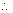IAB =APC [Corresponding angles]

ButCAI =IAB [Given]PCA =APC

AC = AP

Similarly,

BC = BQ

Now,

PQ = AP + AB + BQ

= AC + AB + BC

= Perimeter of ABCQuestion 21

Sides AB and AC of a triangle ABC are equal. BC is produced through C upto a point D such that AC = CD. D and A are joined and produced upto point E. If angle BAE = 108o; find angle ADB.Solution 21

In ABD,

1080 =3 +ADB

But AB = AC

3 = 21080 =2 +ADB ……(i)

Now,

InACD,2=1+ADB

But AC = CD1 =ADB2 =ADB +ADB2 = 2ADB

Putting this value in (i)

1080 = 2ADB +ADB

3ADB = 1080ADB = 360Question 22

The given figure shows an equilateral triangle ABC with each side 15 cm. Also, DE//BC, DF//AC and EG//AB.

If DE + DF + EG = 20 cm, find FG.

Solution 22

Question 23

If all the three altitudes of a triangle are equal, the triangle is equilateral. Prove it.Solution 23

In right BEC and BFC,

BE = CF[Given]

BC = BC[Common]

BEC = BFC[each = 900]

Therefore, ABC is an equilateral triangle.Question 24

In a ABC, the internal bisector of angle A meets opposite side BC at point D. Through vertex C, line CE is drawn parallel to DA which meets BA produced at point E. Show that ACE is isosceles.Solution 24

DA || CE[Given]

[Corresponding angles]

[Alternate angles]

But [ AD is the bisector of A]

From (i), (ii) and (iii)

AC = AE

ACE is an isosceles triangle.Question 25

In triangle ABC, bisector of angle BAC meets opposite side BC at point D. If BD = CD, prove that ABC is isosceles.Solution 25

Hence, ABC is an isosceles triangle.Question 26

In ABC, D is point on BC such that AB = AD = BD = DC. Show that:

ADC: C = 4: 1.Solution 26

Since AB = AD = BD

is an equilateral triangle.

Again in

Question 27

Using the information given in each of the following figures, find the values of a and b.

[Given: CE = AC]

Solution 27

(i)

(ii)

error: Content is protected !!